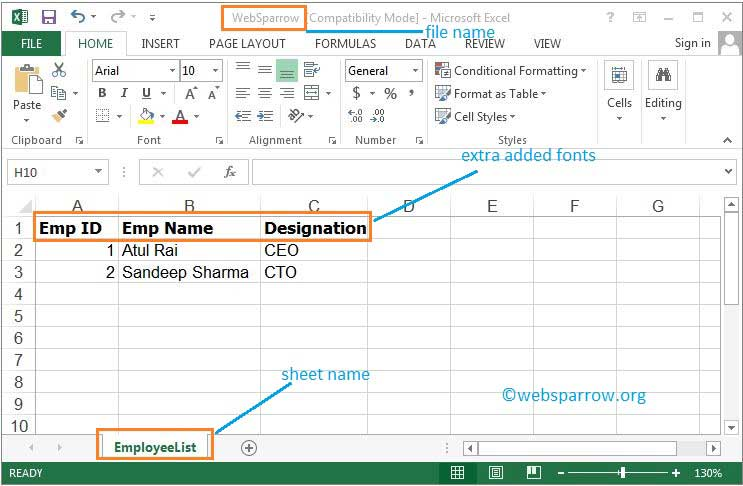# How to Read and Write Excel file in Java

In this tutorial, we will explain How to Read and Write Excel file in Java using `JExcel API`. Let’s see the step by step first we need to create an Excel Workbook and the further step is creating Sheet and adding content into the sheet.

Code Snippet for creating a workbook

``````WritableWorkbook writableWorkbook = null;
writableWorkbook = Workbook.createWorkbook(new File("WebSparrow.xls"));``````

Code Snippet for the reading workbook

``````Workbook wb = null;
wb = Workbook.getWorkbook(new File("WebSparrow.xls"));``````

1. jxl.jar

### Write Excel file

Code to create a new Excel file.

WriteExcelExp.java
``````package org.websparrow;

import java.io.File;

import jxl.Workbook;
import jxl.write.Label;
import jxl.write.Number;
import jxl.write.WritableCellFormat;
import jxl.write.WritableFont;
import jxl.write.WritableSheet;
import jxl.write.WritableWorkbook;

public class WriteExcelExp {
public static void main(String[] args) {
WritableWorkbook writableWorkbook = null;
try {
// create file name
writableWorkbook = Workbook.createWorkbook(new File("WebSparrow.xls"));

// create sheet name
WritableSheet writableSheet = writableWorkbook.createSheet("EmployeeList", 0);

WritableCellFormat writableCellFormat = new WritableCellFormat();
WritableFont writableFont = new WritableFont(WritableFont.TAHOMA, 10, WritableFont.BOLD);
writableCellFormat.setFont(writableFont);

Label lbl = new Label(0, 0, "Emp ID", writableCellFormat);

lbl = new Label(1, 0, "Emp Name", writableCellFormat);

lbl = new Label(2, 0, "Designation", writableCellFormat);

// for first emp
Number num = new Number(0, 1, 1);

lbl = new Label(1, 1, "Atul Rai");

lbl = new Label(2, 1, "CEO");
// for second emp
num = new Number(0, 2, 2);

lbl = new Label(1, 2, "Sandeep Sharma");

lbl = new Label(2, 2, "CTO");

// write all above in to workbook and close
writableWorkbook.write();
writableWorkbook.close();
System.out.println("Done");
} catch (Exception e) {
e.printStackTrace();
}
}
}``````

Output:

When you have complied and run the above code, you will get the below output. To check the output go to your file location and open the generated Excel file.Code to read the above Excel file.

``````package org.websparrow;

import java.io.File;

import jxl.Cell;
import jxl.Sheet;
import jxl.Workbook;

public static void main(String[] args) {
Workbook wb = null;
try {
wb = Workbook.getWorkbook(new File("WebSparrow.xls"));
Sheet sheet = wb.getSheet(0);

Cell c1 = sheet.getCell(0, 0);
Cell c3 = sheet.getCell(1, 0);
Cell c5 = sheet.getCell(2, 0);
System.out.println(c1.getContents() + "  " + c3.getContents() + "         " + c5.getContents());

Cell c2 = sheet.getCell(0, 1);
Cell c4 = sheet.getCell(1, 1);
Cell c6 = sheet.getCell(2, 1);
System.out.println(c2.getContents() + "       " + c4.getContents() + "            " + c6.getContents());

Cell c7 = sheet.getCell(0, 2);
Cell c8 = sheet.getCell(1, 2);
Cell c9 = sheet.getCell(2, 2);
System.out.println(c7.getContents() + "       " + c8.getContents() + "      " + c9.getContents());

// for simple use the below code
/*
Cell c1 = sheet.getCell(0,0);
System.out.print(c1.getContents()+" "); Cell c2=
sheet.getCell(0,1); System.out.println(c2.getContents());

Cell c3 = sheet.getCell(1,0);
System.out.print(c3.getContents()+" "); Cell c4=
sheet.getCell(1,1); System.out.println(c4.getContents());

Cell c5 = sheet.getCell(2,0);
System.out.print(c5.getContents()+" "); Cell c6=
sheet.getCell(2,1); System.out.println(c6.getContents());
*/

wb.close();
} catch (Exception e) {
e.printStackTrace();
}
}
}``````

Output:

``````Emp ID  Emp Name         Designation
1       Atul Rai            CEO
2       Sandeep Sharma      CTO``````